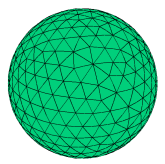﻿ Sample Problems > Usage > 3D_domains > 3d_sphere

3d_sphere

Navigation:  Sample Problems > Usage > 3D_domains >

3d_sphere{ 3D_SPHERE.PDE

This problem considers the construction of a spherical domain in 3D.

The heat equation is  Div(-K*grad(U)) = h, wth U the temperature and

h the volume heat source.

A sphere with uniform heat source h will generate a total amount of heat

H = (4/3)*Pi*R^3*h, from which  h = 3*H/(4*Pi*R^3).

The normal flux at the surface will be Fnormal = -K*grad(U) <dot> Normal,

where Normal is the surface-normal unit vector.  On the sphere, the unit

normal is [x/R,y/R,z/R].

At the surface, the flux will be uniform, so the surface integral of flux is

or  normal(-K*grad(u)) = H/(4*pi*R^2)  =  R*h/3.

 In the following, we set R=1 and H = 1, from which    h = 3/(4*pi)    normal(-k*grad(u)) = 1/(4*pi) }   title '3D Sphere'   coordinates    cartesian3   variables    u   definitions    K = 0.1   { conductivity }    R0 = 1   { radius }    H0 = 1   { total heat }   { volume heat source }    heat =3*H0/(4*pi*R0^3)                     equations    U: div(K*grad(u)) + heat   = 0extrusion

surface z = -SPHERE ((0,0,0),R0)       { the bottom hemisphere }

surface z = SPHERE ((0,0,0),R0)       { the top hemisphere }

boundaries

surface 1 value(u) = 0     { fixed value on sphere surfaces }

surface 2 value(u) = 0

Region 1

start(R0,0)

arc(center=0,0) angle=360

plots

grid(x,y,z)

grid(x,z) on y=0

contour(u) on x=0

contour(-4*pi*k*(x*dx(u)+y*dy(u)+z*dz(u))/sqrt(x^2+y^2+z^2)) on x=0 as "normal flux"

contour(-4*pi*k*(x*dx(u)+y*dy(u)+z*dz(u))/sqrt(x^2+y^2+z^2)) on y=0 as "normal flux"

contour(4*pi*normal(-k*grad(u))) on surface 1 as "4*pi*Normal Flux=1" { bottom surface }

contour(4*pi*normal(-k*grad(u))) on surface 2 as "4*pi*Normal Flux=1" { top surface }

surface(4*pi*normal(-k*grad(u))) on surface 1 as "4*pi*Normal Flux=1" { bottom surface }

surface(4*pi*normal(-k*grad(u))) on surface 2 as "4*pi*Normal Flux=1" { top surface }

summary

report(sintegral(normal(-k*grad(u)),1)) as "Bottom current :: 0.5 "

report(sintegral(normal(-k*grad(u)),2)) as "Top current :: 0.5 "

report(vintegral(heat)) as "Total heat :: 1"

report(sintegral(normal(-k*grad(u)))) as "Total Flux :: 1"

end